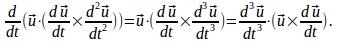## Identity For Derivative of Dot Product of a Curve With Cross Product of First and Second Derivatives

Theorem

If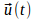is a curve with paprameter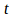then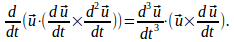Proof

The left hand side can be expanded to give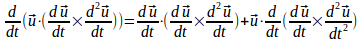The first term on the right above is zero since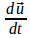is perpendicular to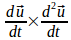and so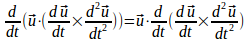Hence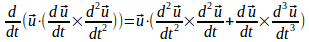but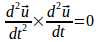so